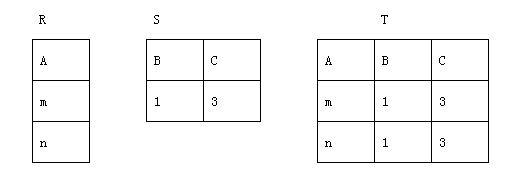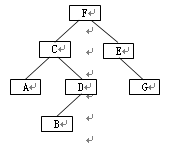• # 全国计算机等级考试二级笔试样卷C语言程序设计

发布时间：2009-03-03                    来源：教育部考试中心

一、选择题（（1）～（10）、（21）～（40）每题2分，（11）～（20）每题2分，共70分）

下列各题A）、B）、C）、D）四个选项中，只有一个选项是正确的，请将正确选项涂写在答题卡相应位置上，答在试卷上不得分。

（1）下列选项中不符合良好程序设计风格的是

A） 源程序要文档化      B） 数据说明的次序要规范化

C） 避免滥用goto语句   D） 模块设计要保证高耦合、高内聚

（2）从工程管理角度，软件设计一般分为两步完成，它们是

A） 概要设计与详细设计  B） 数据设计与接口设计

C） 软件结构设计与数据设计  D） 过程设计与数据设计

（3）下列选项中不属于软件生命周期开发阶段任务的是

A）软件测试     B）概要设计 C）软件维护 D）详细设计

（4）在数据库系统中，用户所见的数据模式为

A） 概念模式    B）外模式  C）内模式   D）物理模式

（5）数据库设计的四个阶段是：需求分析、概念设计、逻辑设计和

A） 编码设计    B） 测试阶段    C）运行阶段 D）物理设计

（6）设有如下三个关系表下列操作中正确的是

A）T＝R∩S      B）T＝R∪S

C）T＝R×S      D）T＝R/S

（7）下列叙述中正确的是

A）一个算法的空间复杂度大，则其时间复杂度也必定大

B）一个算法的空间复杂度大，则其时间复杂度必定小

C）一个算法的时间复杂度大，则其空间复杂度必定小

D）上述三种说法都不对

（8）在长度为64的有序线性表中进行顺序查找，最坏情况下需要比较的次数为

A）63   B）64   C）6    D）7

（9）数据库技术的根本目标是要解决数据的

A）存储问题 B）共享问题 C）安全问题 D）保护问题

（10）对下列二叉树进行中序遍历的结果是

A）ACBDFEG  B）ACBDFGE  C）ABDCGEF  D）FCADBEG

（11）下列叙述中错误的是

A）一个C语言程序只能实现一种算法

B）C程序可以由多个程序文件组成

C）C程序可以由一个或多个函数组成

D）一个C函数可以单独作为一个C程序文件存在

（12）下列叙述中正确的是

A）每个C程序文件中都必须要有一个main()函数

B）在C程序中main()函数的位置是固定的

C）C程序中所有函数之间都可以相互调用，与函数所在位置无关

D）在C程序的函数中不能定义另一个函数

（13）下列定义变量的语句中错误的是

A）int  _int;       B）double  int_;      C）char  For;            D）float  US\$;

（14）若变量x、y已正确定义并赋值，以下符合C语言语法的表达式是

A）++x,y=x--        B）x+1=y          C）x=x+10=x+y         D）double(x)/10

（15）以下关于逻辑运算符两侧运算对象的叙述中正确的是

A）只能是整数0或1                B）只能是整数0或非0整数

C）可以是结构体类型的数据         D）可以是任意合法的表达式

（16）若有定义int  x,y; 并已正确给变量赋值，则以下选项中与表达式(x-y)?(x++) : (y++)中的条件表达式(x-y) 等价的是

A）(x-y>0)      B）(x-y<0)        C）(x-y<0||x-y>0)   D）(x-y==0)

（17）有以下程序

main()

{ int  x, y, z;

x=y=1;

z=x++,y++,++y;

printf("%d,%d,%d\n",x,y,z);

}

程序运行后的输出结果是

A）2,3,3            B）2,3,2              C）2,3,1          D）2,2,1

（18）设有定义：int  a;    float  b;    执行 scanf("%2d%f",&a,&b); 语句时，若从键盘输入876  543.0<回车>，a和b的值分别是

A）876和543.000000               B）87和6.000000

C）87和543.000000                D）76和543.000000

（19）有以下程序

main()

{ int  a=0, b=0;

a=10;                     /*  给a赋值

b=20;                         给b赋值     */

printf("a+b=%d\n",a+b);   /*  输出计算结果 */

}

程序运行后的输出结果是

A）a+b=10           B）a+b=30       C）30          D）出错

（20）在嵌套使用if语句时，C语言规定else总是

A）和之前与其具有相同缩进位置的if配对

B）和之前与其最近的if配对

C）和之前与其最近的且不带else的if配对

D）和之前的第一个if配对

（21）下列叙述中正确的是

A）break语句只能用于switch语句

B）在switch语句中必须使用default

C）break语句必须与switch语句中的case配对使用

D）在switch语句中，不一定使用break语句

（22）有以下程序

main()

{ int  k=5;

while(--k)  printf("%d",k -= 3);

printf("\n");

}

执行后的输出结果是

A）1           B）2            C）4              D）死循环

（23）有以下程序

main()

{ int  i;

for(i=1; i<=40; i++)

{ if(i++%5==0)

if(++i%8==0)  printf("%d ",i);

}

printf("\n");

}

执行后的输出结果是

A）5            B）24             C）32             D）40

（24）以下选项中，值为1的表达式是

A）1 –'0'          B）1 - '\0'       C）'1' -0         D）'\0' - '0'

（25）有以下程序

fun(int  x, int  y){ return (x+y); }

main()

{ int  a=1, b=2, c=3, sum;

sum=fun((a++,b++,a+b),c++);

printf("%d\n",sum);

}

执行后的输出结果是

A）6            B）7             C）8            D）9

（26）有以下程序

main()

{  char  s[]="abcde";

s+=2;

printf("%d\n",s);

}

执行后的结果是

A）输出字符a的ASCII码         B）输出字符c的ASCII码

C）输出字符c                    D）程序出错

（27）有以下程序

fun(int  x, int  y)

{ static int  m=0, i=2;

i+=m+1;    m=i+x+y;    return m;

}

main()

{ int  j=1, m=1, k;

k=fun(j,m);    printf("%d,",k);

k=fun(j,m);    printf("%d\n",k);

}

执行后的输出结果是

A）5, 5         B）5, 11            C）11, 11       D）11, 5

（28）有以下程序

fun(int  x)

{ int  p;

if(x==0||x==1)  return(3);

p=x-fun(x-2);

return p;

}

main()

{  printf("%d\n",fun(7)); }

执行后的输出结果是

A）7            B）3            C）2            D）0

（29）在16位编译系统上，若有定义int  a[]={10,20,30}, *p=&a;，当执行p++;后，下列说法错误的是

A）p向高地址移了一个字节        B）p向高地址移了一个存储单元

C）p向高地址移了两个字节        D）p与a+1等价

（30）有以下程序

main()

{ int  a=1, b=3, c=5;

int  *p1=&a, *p2=&b, *p=&c;

*p =*p1*(*p2);

printf("%d\n",c);

}

执行后的输出结果是

A）1            B）2            C）3            D）4

（31）若有定义：int w; ，则以下不能正确表示该数组元素的表达式是

A）*(*w+3)      B）*(w+1)        C）*(*(w+1))        D）*(&w+1)

（32）若有以下函数首部

int  fun(double  x, int  *n)

则下面针对此函数的函数声明语句中正确的是

A）int  fun(double x, int *n);      B）int  fun(double  , int );

C）int  fun(double *x, int n);      D）int  fun(double *,  int *);

（33）有以下程序

void change(int k[ ]){ k=k; }

main()

{ int  x={1,2,3,4,5,6,7,8,9,10},n=0;

while( n<=4 )  { change( &x[n]) ; n++; }

for(n=0; n<5; n++)  printf("%d ",x[n]);

printf("\n");

}

程序运行后输出的结果是

A）6 7 8 9 10       B）1 3 5 7 9       C）1 2 3 4 5       D）6 2 3 4 5

（34）有以下程序

main()

{ int  x={0}, i;

for(i=0; i<3; i++)    scanf("%d",x[i]);

printf("%3d%3d%3d\n",x,x,x);

}

若运行时输入：2 4 6<回车>，则输出结果为

A）2  0  0      B）2  0  4      C）2  4  0      D）2  4  6

（35）有以下程序

int add( int  a,int  b){ return (a+b);  }

main()

{ int  k, (*f)(), a=5,b=10;

f=add;

…

}

则以下函数调用语句错误的是

A）k=(*f)(a,b);                 B）k=add(a,b);

C）k= *f(a,b);                      D）k=f(a,b);

（36）有以下程序

#include

main( int  argc, char  *argv[ ])

{ int  i=1,n=0;

while (i

printf("%d\n",n);

}

该程序生成的可执行文件名为：proc.exe。若运行时输入命令行：

proc  123  45  67

则程序的输出结果是

A）3            B）5            C）7            D）11

（37）有以下程序

# include

# define    N    5

# define    M    N+1

# define    f(x)   (x*M)

main()

{ int  i1, i2;

i1 = f(2) ;

i2 = f(1+1) ;

printf("%d  %d\n", i1, i2);

}

程序的运行结果是

A）12  12       B）11  7        C）11  11       D）12  7

（38）有以下结构体说明、变量定义和赋值语句

struct STD

{ char  name;

int  age;

char  sex;

} s,*ps;

ps=&s;

则以下scanf函数调用语句中错误引用结构体变量成员的是

A）scanf("%s",s.name);          B）scanf("%d",&s.age);

C）scanf("%c",&(ps->sex));         D）scanf("%d",ps->age);

（39）若有以下定义和语句

union data

{  int  i;   char  c;   float  f; } x;

int  y;

则以下语句正确的是

A）x=10.5;      B）x.c=101;     C）y=x;         D）printf("%d\n",x);

（40）有以下程序

#include

main()

{ FILE  *fp;    int  i;

char  ch[]="abcd",t;

fp=fopen("abc.dat","wb+");

for(i=0; i<4; i++) fwrite(&ch[i],1,1,fp);

fseek(fp,-2L,SEEK_END);

fread(&t,1,1,fp);

fclose(fp);

printf("%c\n",t);

}

程序执行后的输出结果是

A）d            B）c            C）b            D）a

二、填空题（每空2分，共30分）

请将每一个空的正确答案写在答题卡【1】至【15】序号的横线上，答在试卷上不得分。

（1）下列软件系统结构图

的宽度为  【1】  。

（2）  【2】  的任务是诊断和改正程序中的错误。

（3）一个关系表的行称为   【3】   。

（4）按“先进后出”原则组织数据的数据结构是  【4】    。

（5）数据结构分为线性结构和非线性结构，带链的队列属于  【5】   。

（6）设有定义：float  x=123.4567;，则执行以下语句后的输出结果是 【6】

printf("%f\n",(int)(x*100+0.5)/100.0);

（7）以下程序运行后的输出结果是 【7】

main()

{ int  m=011,n=11;

printf("%d  %d\n",++m, n++);

}

（8）以下程序运行后的输出结果是 【8】

main()

{ int  x,a=1,b=2,c=3,d=4;

x=(a   x=(x   x=(d>x) ? x : d;

printf("%d\n",x);

}

（9）有以下程序，若运行时从键盘输入：18,11<回车>，则程序的输出结果是 【9】

main()

{  int  a,b;

printf("Enter a,b:");   scanf("%d,%d",&a,&b);

while(a!=b)

{  while(a>b) a -= b;

while(b>a) b -= a;

}

printf("%3d%3d\n",a,b);

}

（10）以下程序的功能是：将输入的正整数按逆序输出。例如：若输入135则输出531。请填空。

#include

main()

{ int  n,s;

printf("Enter a number : ");   scanf("%d",&n);

printf("Output:  ");

do

{  s=n%10;  printf("%d",s);  【10】 ;  }

while(n!=0);

printf("\n");

}

（11）以下程序中，函数fun的功能是计算x2-2x+6，主函数中将调用fun函数计算：

y1=(x+8)2-2 (x+8)+6

y2=sin2(x)-2sin(x)+6

请填空。

#include   "math.h"

double fun(double  x){ return (x*x-2*x+6); }

main()

{ double  x,y1,y2;

printf("Enter x:");    scanf("%lf",&x);

y1=fun( 【11】 );

y2=fun( 【12】 );

printf("y1=%lf,y2=%lf\n",y1,y2);

}

（12）下面程序的功能是：将N行N列二维数组中每一行的元素进行排序，第0行从小到大排序，第1行从大到小排序，第2行从小到大排序，第3行从大到小排序，例如：

#define    N    4

void sort(int  a[][N])

{ int  i, j, k, t;

for (i=0; i

for (j=0; j

for (k=  【13】 ; k

/*判断行下标是否为偶数来确定按升序或降序来排序*/

if ( 【14】 ? a[i][j]a[i][k])

{  t = a[i][j];

a[i][j]=a[i][k];

a[i][k] = t;

}

}

void outarr(int  a[N][N])

{    ……    }

main()

{ int  aa[N][N]={{2,3,4,1},{8,6,5,7},{11,12,10,9},{15,14,16,13}};

outarr(aa); /*以矩阵的形式输出二维数组*/

sort(aa);

outarr(aa);

}

（13）下面程序的运行结果是：【15】  。

#include

int f(int  a[],int  n)

{ if(n>1)

return a + f(a+1, n-1);

else

return a;

}

main()

{ int  aa={1,2,3,4,5,6,7,8,9,10}, s;

s = f(aa+2,4);    printf("%d\n", s);

}